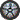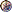### Prof. Paulo Franca - Spring 2000

Introduction

Syllabus

Guidelines for labs

Lab sections

Take quiz & check grades

### Recurrences

#### Purpose of this chapter:

to let you compute the bounds on the running time of a given algorithm when the expression for T(n) is not readily available as a function of n. T(n) is available as a recurrence.

#### 3 methods:  (pages 53 to 64)##### substitution methodguess a bound and use mathematical induction to prove the guess correct##### iteration methodconverts the recurrence into a summation and try to find the solution##### master methodcan only be used for recurrences of the form T(n)=aT(n/b) + f(n). It does not cover all the cases.

#### Recurrences:

The running time is expresssed by a recurrence relation:

Example:

T(n) is:Teta(1) if n=12* T(n/2)+Teta(n) if n>1